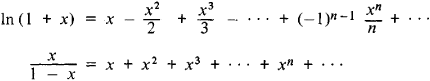# Majorant and Minorant

The following article is from The Great Soviet Encyclopedia (1979). It might be outdated or ideologically biased.

## Majorant and Minorant

(mathematics), two functions the values of the first of which are not less than, and those of the second not greater than, the corresponding values of a given function (for all given values of the independent variable). For example, the function f(x) = x is the majorant for x > — 1 of the function g(x) = In (1 + x) since x ≥ In (1 + x) for all values x > — 1.

For functions that can be represented by a power series, the term “majorant” is often given a more special meaning. In such cases the term denotes the sum of a power series with positive coefficients that are not less than the absolute values of the corresponding coefficients of the given series. If f1(x) is a majorant (in this special sense) of the function g(x), then we write f1(x) » g(x). For example, x/(1 — x) » In (1 + x) sinceIn this (special) sense f(x) = x is no longer the majorant of In (1 + x). The majorants of power series are widely used in the theory of differential equations. For example, the method for the approximate solution of differential equations proposed in 1919 by the Soviet scientist S. A. Chaplygin is based on the use of majorants.

The Great Soviet Encyclopedia, 3rd Edition (1970-1979). © 2010 The Gale Group, Inc. All rights reserved.
Site: Follow: Share:
Open / Close